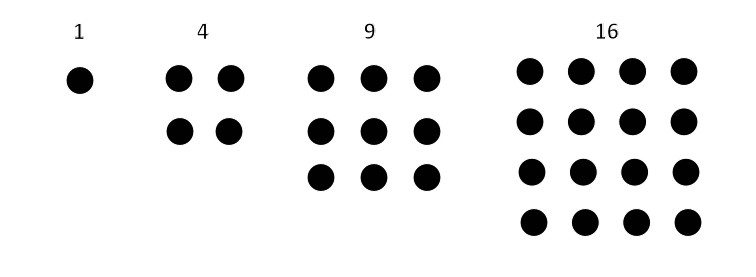created 05/26/03; edited 11/09/2012, 07/09/2016, 04/07/2018

# Chapter 25 Programming Exercises

## Exercise 1 — Log Table

Modify the program that prints out a table of `x` and `ln(x)` so that the table is formatted using HTML. Do this by first printing:

```<html>
<body>
<table>
<tr><th>x</th><th>ln(x)</th></tr>
```

Next, for each iteration of the loop, calculate `x` and `logx` and print the line:

```System.out.println("<tr><td>" + x + "</td><td>" + logx + "</td></tr>" );
```

Finally, print:

```</table>
</body>
</html>
```

Run your program and redirect the output to a disk file.

```C:\Javacode>java LogTable > myLogs.html
```

You should now be able to view your output file with a Web browser. (Find it in your subdirectory and double click on it, or use "File/Open" in your browser's menu.)

Output redirection (which is used here) is further discussed in chapter 70.

## Exercise 2 — Birthday Paradox

How many people do you need at a party for there to be a good chance that two of them have the same birthday?

Say you would like a 50% chance that two of the guests at a party have the same birthday. You want the same month and day, but ignore the year. You don't care what particular month and day as long as two guests happen to have the same.

In a group of two people, the first person can have any of 365 birthdays. The second person must avoid that birthday, so can have any of 364 birthdays. The chances that this happens is 364 out of 365. So probability that two people have different birthdays is

```(365-1)/365
```

A third person that joins the group must avoid the first two birthdays. So the third person can pick any of 363 birthdays. The chances that this happens is 363 out of 365. The probability that three people have different birthdays is the probability that the first two are different times the probability that the third is different:

```((365 - 1)/365)*((365 - 2)/365)
```

Each time a person is added, there is one more birthday to avoid. So

```Probability( no shared birthdays among n people ) = ((365 - 1)/365) * ((365 - 2)/365) * ... * ( (365 - (n-1))/365)
```

Of course, when n is greater than 365 the probability is zero.

Program: Write a program that asks for the number of guests, n, and calculates the probability that they do not share a birthday. You will need a for-loop where each iteration calculates one factor in the above formula. Find the number of guests it takes for there to be more than a 50% chance (probability = 0.50) that none share a birthday (so there is about a 50% chance that two of them do share the same birthday.)

This is called the "birthday paradox" because n is surprisingly small.

## Exercise 3 — Triangle and Square Numbers

A square number is one where that many pebbles can be arranged into a square.So 1, 4, 9, 16, 25, ... are square numbers. A triangle number is one where that many pebbles can be arranged into a triangle, with one pebble at the top, two in the next row, three in the third row, and so on.So 1, 3, 6, 10, 15, ... are triangle numbers. Are there any numbers that are both square and triangle? There is at least one:

```36 = 1+2+3+4+5+6+7+8 = 6*6
```

Are there others? Write a program that finds all integers from one to some upper limit that are both square and triangular.

## Exercise 4 — Monkeys in a Tree

Write a program that prints out this nursery rhyme.

```Five little monkeys swinging in a tree
Teasing Mr Crocodile "you can't catch me"
Along came the crocodile as quiet as can be
and SNAP!

Four little monkeys swinging in a tree
Teasing Mr Crocodile "you can't catch me"
Along came the crocodile as quiet as can be
and SNAP!

Three little monkeys swinging in a tree
Teasing Mr Crocodile "you can't catch me"
Along came the crocodile as quiet as can be
and SNAP!

Two little monkeys swinging in a tree
Teasing Mr Crocodile "you can't catch me"
Along came the crocodile as quiet as can be
and SNAP!

One little monkeys swinging in a tree
Teasing Mr Crocodile "you can't catch me"
Along came the crocodile as quiet as can be
and SNAP!

No little monkeys swinging in a tree
No little monkeys swinging in a tree
```

Of course, use a counting loop. The loop body prints out one verse, modified for the current count. Use if-statements to pick the right word for the number of monkeys.

Google "monkeys in a tree" to find cute you-tube videos of this song.